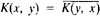# Hermitian Operator

## Hermitian operator

[er′mish·ən ′äp·ə‚rād·ər]
(mathematics)
A linear operator A on vectors in a Hilbert space, such that if x and y are in the range of A then the inner products (Ax,y) and (x,Ay) are equal.
McGraw-Hill Dictionary of Scientific & Technical Terms, 6E, Copyright © 2003 by The McGraw-Hill Companies, Inc.
The following article is from The Great Soviet Encyclopedia (1979). It might be outdated or ideologically biased.

## Hermitian Operator

an infinite-dimensional analogue of the Hermitian linear transformation. A bounded linear operator A in a complex Hilbert space H is said to be Hermitian if for any two vectors x and y in the space the relation (Ax, y) = (x, Ay) holds, where (x, y) is the scalar product of H. Examples of Hermitian operators are integral operators (seeINTEGRAL EQUATIONS) for which the kernel K(x, y) is given in a bounded region and is a continuous function such that; in this case, K(x, y) is called a Hermitian kernel. The concept of Hermitian operators may be extended to unbounded linear operators in a Hilbert space.

Hermitian operators play an important role in quantum mechanics, providing a convenient means of describing mathematically the observable quantities that characterize a physical system.

References in periodicals archive ?
This coalescence is, on principle, different from the well-known degeneration of two eigenstates of a Hermitian operator H.
We say that T [member of] B(E) is a Hermitian operator if T [member of] H(B(E)).
where b is the vector storing the measured data, and the superscript H denotes the Hermitian operator. Due to the ill-posedness of the problem, the above summation is terminated early, say at the index K.
Given a Hermitian operator M on a vector space V with a subspace U [subset or equal to] V, let [pr.sub.U]: V [right arrow] U denote the orthogonal projection, and then denote by [M.sub.U] the Hermitian operator on U given by [M.sub.U] = [pr.sub.U] [omicron] M [omicron] [pr.sub.U].
Some other specific subjects which I found interesting were related to the iterative method for computing the square root for a positive Hermitian operator [6, p.
Taking into account that the Hamiltonian is a Hermitian operator, it is possible to show that for n [not equal to] 1:
Weyl  examined the spectra of all compact perturbations of a hermitian operator on Hilbert space and found in 1909 that their intersection consisted precisely of those points of the spectrum which were not isolated eigenvalues of finite multiplicity.
We show that every Hermitian operator [??] in the spherical symmetric problem ([??] = R-1[??] R) can be written in the form
Observations of the unstable particle can be also described in the quantum-logical language of yes-no questions, like "Do we see the unstable particle?" and an observable, which can take two values 1 or 0, corresponding to the possible answers "yes" or "no." Obviously, the Hermitian operator of this observable is the projection T introduced above.
Since the Hamiltonian is a Hermitian operator, one concludes that if the Hilbert space basis yields a non-diagonal Hamiltonian matrix then the lowest eigenvalue "favors" eigenfunctions that are a linear combination of the Hilbert space basis functions.
H.Weyl  examined the spectra of all compact perturbations of a hermitian operator on Hilbert space and found in 1909 that their intersection consisted precisely of those points of the spectrum which were not isolated eigenvalues of finite multiplicity.
The difference in the resolvents of two self-adjoint extensions of the non-densely defined Hermitian operator A = -[Laplacian operator] (when restricted to an appropriate subspace of [L.sup.2](0,x)) is studied by explicitly constructing a Green's function solution.

Site: Follow: Share:
Open / Close